# High School Chemistry : Help with Buffers

## Example Questions

← Previous 1

### Example Question #1 : Buffers

Which of the following would best buffer a solution from a pH of 4 to 6?

Possible Answers:

Hydrocyanic acid (pKa = 9.2)

Uric acid (pKa = 3.9)

Formic acid (pKa = 3.7)

Carbonic acid (pKa = 6.3)

Acetic acid (pKa = 4.7)

Correct answer:

Acetic acid (pKa = 4.7)

Explanation:

A weak acid/base best buffers about 1 pH point above and below its pKa. The pKA closest to the middle of 4 and 6 (so want as close to 5) is acetic acid at 4.7.

### Example Question #1 : Help With Buffers

Which of the following solutions has the greatest buffering capacity?

Possible Answers:

1M Acetic Acid

3M Rubidium Hydroxide

2M Formic Acid

4M Nitric Acid

Correct answer:

2M Formic Acid

Explanation:

Nitric Acid is a strong acid and can't buffer. Rubidium Hydroxide is a strong base and thus can't buffer. Of the remaining, both are weak acids, but the one with a greater concentration has a greater buffering capacity.

### Example Question #4 : Buffers

To create a buffer solution, you can use a weak acid and                       .

Possible Answers:

its conjugate acid

another weak acid

its conjugate base

a strong base

Correct answer:

its conjugate base

Explanation:

The definition of a buffer solution is that it contains a weak acid and its conjugate base, or a weak base and its conjugate acid. Since we are starting with a weak acid in this case, we need its conjugate base.

### Example Question #5 : Buffers

Which of the following will increase the pH of an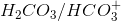buffer solution?

I. Removing carbonic acid

II. Adding sodium bicarbonate

Possible Answers:

Both I and II

I only

Neither of these options

II only

Correct answer:

Both I and II

Explanation:

To answer this question we need to look at the reaction below: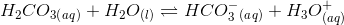An increase in the pH will result in a decrease in the concentration of hydrogen ions (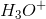). Using Le Chatelier’s principle we can find out which answer choices will decrease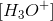.

Removing carbonic acid will decrease the concentration of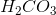. To maintain equilibrium, the reaction will shift to the left and make more reactants from products; therefore, there will be a decrease in theand an increase in pH.

Recall that salts like sodium bicarbonate, or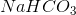, will dissociate in water and form ions. Sodium bicarbonate will form sodium (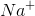) and bicarbonate (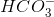) ions. This side reaction will result in an increase in the bicarbonate ion concentration. Le Chatelier’s principle will shift the equilibrium of the given reaction to the left and, therefore, decrease the. Adding sodium bicarbonate will increase the pH.

### Example Question #6 : Buffers

Which of the following combinations cannot be used to produce a buffer solution?

Possible Answers: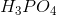and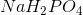and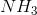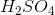and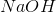andCorrect answer:andExplanation:

Buffer solutions can be made via two methods. The first method involves adding equal amounts of a weak acid and a salt of its weak conjugate base (or vice versa). The second methods involves adding a weak acid and a half equivalent of a strong base (or vice versa).is a weak acid andis a salt of its weak conjugate base; therefore, this can form a buffer.is a weak base and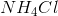is a salt of its weak conjugate acid; this can also form a buffer. Note that this is the converse of the first method (weak base with salt of weak acid), but it can still form a buffer solution.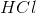is a strong acid andis a weak base; therefore, addingand a half equivalent ofwill create a buffer solution. This is the converse of the second method (adding a weak base to a half equivalent of strong acid).andare both strong reagents (acid and base, respectively); therefore, they cannot form a buffer solution.

### Example Question #8 : Buffers

A researcher is trying to make a buffer solution from a weak acid and its weak conjugate base. The pKa of the acid is 5.9 and the desired pH of the buffer solution is 3.5. Which of the following is the best way to make this buffer solution?

Possible Answers:

The researcher cannot use this weak acid to make the desired buffer solution

Add a 1:1 ratio of the weak acid to its weak conjugate base

Add a 1:1 ratio of the weak acid to a strong base

Add a 2:1 ratio of the weak acid to a strong base

Correct answer:

The researcher cannot use this weak acid to make the desired buffer solution

Explanation:

One way to make a buffer is by adding equal amounts of a weak acid to its weak conjugate base. For example, you can add 1M acetic acid to 1M acetate to create a buffer solution (note that both acetic acid and its conjugate base (acetate) are weak). However, when using this method you have to remember that the desired pH of the buffer solution has to equal the pKa of the weak acid. The question states that the pKa of the acid is 5.9 and the desired pH of the buffer is 3.5; therefore, it is not possible to make the buffer with the given acid. The researcher would have to find another acid that has a pKa near 3.5.

### Example Question #1 : Help With Buffers

Which of the following would be most useful as a buffer?

Possible Answers:

Water

A solution of sodium chloride and sodium hydroxide

A solution of ammonia and ammonium chloride

A solution of carbonic acid and sodium chloride

Potassium hydroxide

Correct answer:

A solution of ammonia and ammonium chloride

Explanation:

A buffer must contain either a weak base and its salt or a weak acid and its salt. A mixture of ammonia and ammonium chloride is an example of the first case, since ammonia, NH3, is a weak base and ammonium chloride, NH4Cl, contains its salt.

Though autoionization of water produces small amounts of H3O+ and OH-, each conjugate salts of H2O, they exist in such small amounts as to make any buffering effects negligible.

### Example Question #1 : Buffers

The pH of a buffered solution is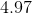. What is the approximate ratio of the concentration of acid to conjugate base if the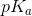of the acid is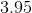?

Possible Answers: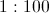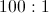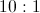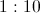Correct answer:Explanation:

In a buffered solution, when the concentrations of acid and conjugate base are equal, we know the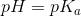.

This is derived from the Henderson-Hasselbalch equation: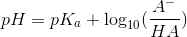.

When concentrations are equal, the log ofis, and.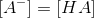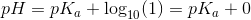In our question, the pH is approximately one unit greater than the.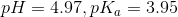Because of this, we know that the log of the two concentrations must be equal to one.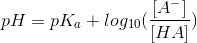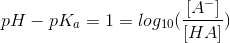The log ofis, and therefore the conjugate base must be ten times greater than the acid; therefore the ratio of acid to base is approximately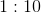.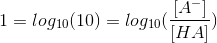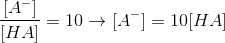We can quickly narrow this question to two possible answers, since the buffered solution is more basic than the, and thus we would expect there to be a greater concentration of base than acid in the solution.

### Example Question #3 : Help With Buffers

Acids and bases can be described in three principal ways. The Arrhenius definition is the most restrictive. It limits acids and bases to species that donate protons and hydroxide ions in solution, respectively. Examples of such acids include HCl and HBr, while KOH and NaOH are examples of bases. When in aqueous solution, these acids proceed to an equilibrium state through a dissociation reaction.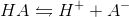All of the bases proceed in a similar fashion.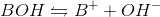The Brønsted-Lowry definition of an acid is a more inclusive approach. All Arrhenius acids and bases are also Brønsted-Lowry acids and bases, but the converse is not true. Brønsted-Lowry acids still reach equilibrium through the same dissociation reaction as Arrhenius acids, but the acid character is defined by different parameters. The Brønsted-Lowry definition considers bases to be hydroxide donors, like the Arrhenius definition, but also includes conjugate bases such as the A- in the above reaction. In the reverse reaction, A- accepts the proton to regenerate HA. The Brønsted-Lowry definition thus defines bases as proton acceptors, and acids as proton donors.

A scientist is making a buffer to maintain the pH of a solution at about 6. Which of the following describes the best composition of the solution?

Possible Answers:

A larger amount of acid , with some conjugate base, where the acid has a pKa of 5.9.

About equal amounts of acid and conjugate base, where the acid has a pKa of 6.9.

A larger amount of acid , with some conjugate base, where the acid has a pKa of 4.9.

About equal amounts of acid and conjugate base, where the acid has a pKa of 5.9.

A larger amount of acid , with some conjugate base, where the acid has a pKa of 6.9.

Correct answer:

About equal amounts of acid and conjugate base, where the acid has a pKa of 5.9.

Explanation:

A buffer is best made of equal and copious amounts of an acid and its conjugate base. The acid must have a pKa as close as possible to the pH desired. For this question, the desired pH is 6. The best option will have a pKa of about 5.9.

Adding larger amounts of the acid than the base will skew the equilibrium in favor of the acid, increasing the overall proton concentration, and limiting the buffer ability to absorb more proton addition without changing pH significantly.

### Example Question #1 : Help With Buffers

Which of the following pairs cannot constitute a buffer system?

Possible Answers: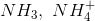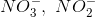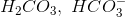Correct answer:Explanation:

A buffer system is composed of one of the following scenarios:

1. A weak base with its conjugate acid.

2. A weak acid with its conjugate base.

The only option that does not qualify is the nitrate, nitrite pair. A difference of one oxygen does not make a buffer system.

← Previous 1

### All High School Chemistry Resources